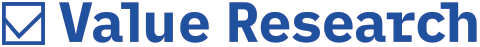# Measuring risk sensibly

##### Using a measure of risk-adjusted return that takes into account only negative deviations from the mean can be illuminating

Portfolio management focuses on risk-adjusted returns rather than on absolute returns. Higher returns are associated with more risk. If things go wrong, as they do sometimes, investors must know the potential downside. Risk-adjustment makes it apparent when higher absolute returns are not worth aiming for because the extra risk is too high.

The problem arises with the standard methods of measuring risk. The commonest measure is based on standard deviation (SD) of returns. To calculate SD, the difference between each return and the mean return is calculated. This is squared (to remove negatives), and averaged to find variance. The SD is the square root of variance. For example, with the series 1, 2, 3, the mean is 2, variance is 0.667 {average of ((1-2)^2+ (2-2)^2 +(3-2)^2)} and the SD is 0.816 (square root of 0.667).

SD and variance gauge dispersion from the mean. For a normal distribution where the mean is in the middle, about 68 per cent of returns fall within one standard deviation (mean +/- SD). About 95 per cent of returns fall within two SDs (mean +/- 2SD). Over 99 per cent of all returns fall within three SDs (mean +/-3SD).

Understanding how much a given return may vary from the mean return is useful and an excellent measure of volatility. But volatility doesn't equal risk. There is no distinction between positive difference and negative difference mathematically. An investor would be pleased with a larger positive difference but SD accounts this as a risk. So it gives a skewed picture and affects risk-adjustment measures.

The most commonly used risk-adjustment measure is the Sharpe Ratio. This assigns a risk-free return, such as treasury bill yields or fixed deposit interest rates. The risk-free return is subtracted from the mean return of the target portfolio and then divided by the SD.

Using only downside variance
One refinement of Sharpe is the Sortino Ratio, which uses only downside variance. Sortino ignores returns higher than the mean (or the risk-free rate, if that is the target). The rest of the calculation is similar. By focussing on downside, Sortino uses semi-variance and semi-standard deviation. The semi-standard deviation is closer to the intuitive definition of risk.

These two ratios - Sortino and Sharpe - may be quite different in a healthy economy, where the long-term stock market return is positive. In such cases, the semi-variance will generally be lower than the variance because there will be strongly positive returns that are excluded when calculating semi-variance. Hence, the Sortino ratio will be higher than the Sharpe ratio.

It may not make that much difference in cases of assets like forex, for example, since the asset under consideration doesn't have a long-bias. This is because any forex position is automatically long in one currency and short in another.

As an example, I calculated daily, month-on-month and year-on-year Nifty returns from January 2000 to June 2006, annualising the last six months or YoY. The results are interesting. At YoY level, the average Nifty return is 17.7 per cent for this 126-month period. The standard deviation is 40.9 per cent. Assuming a risk-free rate of 8 per cent, the YoY SD is 0.23, which is nothing special.

When we calculate the semi-variance, however, the YoY semi-standard deviation drops to 33.4 per cent, revealing considerable long bias. The YoY Sortino Ratio is 0.53, which is a lot better than the Sharpe. The Sortino suggests you can expect roughly half unit of return for every extra unit of risk.

At the monthly level, the situation is different. The average MoM return is 1.3 per cent while the SD is 8.8 per cent and the semi-variance is 9.3. The Sortino (0.065) is therefore lower than the Sharpe (0.07) at the MoM level. At the daily level, the Sortino ratio is 0.036 while the Sharpe is slightly lower at 0.034.

Key inferences
One can draw certain inferences. One is that the Sharpe ratio itself tells us that a longer term period of investment offers better risk-adjusted returns and that's confirmed by the Sortino. Another is that there is considerable positive bias to the YoY Nifty returns whereas there is actually some negative bias at the MoM level. The second conclusion is a little surprising but there have actually been quite a few months in this 10.5-year period where there have been large MoM losses. At DoD level, the Sortino again outscores Sharpe but only at third decimal point.

Obviously, the results would be different for a different portfolio and for mutual funds that don't track the Nifty closely, it could be very different. But using both semi-variance as well as variance as risk-adjustment measures is illuminating for a portfolio theorist.